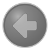# Pyxplot

## Examples - Labelled trianglesDownload this example:

Using the polygon command and Pyxplot's maths environment to construct triangles

In this example, we make a subroutine to draw labelled diagrams of the interior angles of triangles, taking as its inputs the lengths of the three sides of the triangle to be drawn and the position of its lower-left corner. The subroutine calculates the positions of the three vertices of the triangle and then labels them. We use Pyxplot's automatic handling of physical units to generate the LaTeX strings required to label the side lengths in centimetres and the angles in degrees. We use Pyxplot's arc command to draw angle symbols in the three corners of a triangle.

### Script

```set unit angle nodimensionless
set unit of length cm # Display lengths in cm
set unit of angle degree # Display angles in degrees
set numeric sigfig 3 display latex # Correct to 3 significant figure
cm  = unit(cm) # Shorthand to save space
deg = unit(deg)

turn(a) = matrix( [cos(a),-sin(a)], \
[sin(a), cos(a)]  )

# Define subroutine for drawing triangles
subroutine triangleDraw(B,AB,AC,BC)
{
# Use cosine rule to find interior angles
ABC = acos((AB**2 + BC**2 - AC**2) / (2*AB*BC))
BCA = acos((BC**2 + AC**2 - AB**2) / (2*BC*AC))
CAB = acos((AC**2 + AB**2 - BC**2) / (2*AC*AB))

# Positions of three corners of triangle
C = B + vector(BC,0*cm)
A = B + turn(ABC)*vector(AB,0*cm)

# Draw triangle
polygon [A,B,C]

# Draw angle symbols

# Label lengths of sides
textGap = 0.1*cm
text "%s"%(BC) at (B+C)/2 gap textGap hal c val t
text "%s"%(AB) at (A+B)/2 gap textGap rot  ABC hal c val b
text "%s"%(AC) at (A+C)/2 gap textGap rot -BCA hal c val b

# Label angles
text "%s"%CAB at A+turn(-90*deg+ABC-BCA)*arcRad2 hal c val t
text "%s"%ABC at B+turn(          ABC/2)*arcRad2 hal l val c
text "%s"%BCA at C+turn(180*deg - BCA/2)*arcRad2 hal r val c

# Label points ABC
text "A" at A gap textGap hal c val b
text "B" at B gap textGap hal r val c
text "C" at C gap textGap hal l val c
}

# Display diagram with three triangles
set multiplot ; set nodisplay
call triangleDraw(vector([2.8,3.2])*cm, 3*cm, 4*cm, 4*cm)
call triangleDraw(vector([0.0,0.0])*cm, 3*cm, 4*cm, 5*cm)
call triangleDraw(vector([6.5,0.0])*cm, 3*cm, 3*cm, 3*cm)
set display ; refresh

```